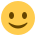#### Howdy, Stranger!

It looks like you're new here. If you want to get involved, click one of these buttons!

Supported by

# Jitter from exponential distribution

Dear OS communityI'm trying to create a jitter randomize according to a non-symmetric exponential distribution (truncated at 4000 msc). I need the jitter to randomize between 500 msc till 4000 msc and average of 1000 msc.

Any ideas?

Thanks
SH

• edited April 2018

Hmm, no idea what a jitter time is! I can maybe help a bit with the distribution. The below will generate a truncated exponential distribution between 0-3500ms using the inverse cdf method, then add on 500ms. This almost gives you what you want. The mean will be a little shy of 1000 (990 something) because you are cutting a tiny bit of the tail off.

``````import numpy as np
#0.5 is 500ms, desired mean before we add on the initial 500
p= 1 - np.exp(-1/0.5)
#generate uniform random numbers
r=np.random.rand( 100000)
# N is the highest delay allowed (in seconds). 3500ms until we add on initial 500 ms at theend
N=3.5
#x1000 to return in milliseconds as opensesame expects.
gen_trunc=np.log(1-r*(1-(1-p)**N))/np.log(1-p) * 1000
#add on 500 to get max 4000 and mean (almost) 1000
jitter = gen_trunc + 500
print np.mean(jitter)

import matplotlib.pyplot as plt
bins=np.arange(0,4500,1)
plt.hist(jitter,bins=bins,alpha=.8)
plt.show()
``````
• Wow. Thank you so much!

How can I randomly sample the function you've created?

• Sorry for slow reply, the variable jitter is a big array of random numbers drawn from the distribution you wanted, so just take as many numbers out as you need starting from 1 to 100,000

cheers

• I'll try that.. Thanks!!!!!